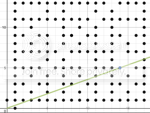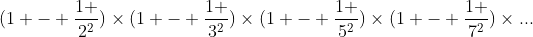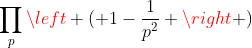0

## Math Out in the Field (Part 2)

Published on Thursday, December 06, 2012 in , , , ,This post picks up from the previous post, in which I explored an integer lattice using Desmos.

Today, we'll keep exploring the integer lattice, and see what other surprises it offers!

As before, the 20 by 20 integer lattice is here (opens in new window), and the 10 by 10 integer lattice is here (opens in new window). The latter functions better on mobile devices. Clicking on the options button in the upper right corner, then selecting Projector mode is also recommended.

PRIMES AGAIN

We left off in the last post talking about prime numbers, integers (whole numbers) that are only even divisible by 1 and themselves. This seemed like a good place to continue the discussion.

If you take a look at the column (3,y), or the row (x,3) for that matter, you'll notice that only every 3rd dot isn't visible. This shouldn't be surprising by now, since we know from the previous post that only the dots that are visible are the ones that are coprime (their only common divisor is 1).

Similarly, with (x,2) and (2,y) only every 2nd one isn't visible, with (x,5) and (5,y) only every 5th one isn't visible, and so on. It's a simple pattern, but one that can help you easily pick out the prime numbers in even very large integer lattices.

DIAGONAL PRIMES

For the next couple of surprises, I've set up a 2nd set of lattices. Here's the new 20 by 20 lattice (opens in a new window), and this link goes to the new 10 by 10 lattice (opens in a new window).

In either of these, start clicking on the Xs in the icons of the formulas until you get down to the next set of text instructions. When you do this, diagonal purple lines will appear. First, notice that diagonals not highlighted by purple lines have missing dots, while the highlighted diagonals have unbroken lines of dots (at least when they're not crossing an axis or going off the other edge of the grid).

What's so special about these purple lines? In each (x, y) pair, the sum of x and y add up to prime numbers! For example, in the coordinates (1,6), (2,5), (3,4), (4,3), (5,2), and (6,1), all the pairs add up to 7, and they're all visible! These are the dots highlight with the formula y=7-x, where x is greater than 0.

It turns out that, regardless of the size of the integer lattice you use, coordinate pairs whose sum is a prime number will always have an unbroken line of visible dots (again, as long as neither coordinate is 0). But what exactly does this mean?

From the previous post, we know that the dots on the lattice are only visible when their coordinate pair is coprime. So, we can determine from this that any two non-zero positive integers whose sum is a prime number must be coprime to each other! Take another look at all the pairs above that total 7, and you'll see that none of those pairs can be reduced!

This surprising discovery, done with a grid and simple arithmetic, is part of the reason I find the integer lattice so fascinating.

ARE THERE LINES THAT WILL NEVER CROSS ANY DOT?

Imagine that the integer lattice we've been using is extended infinitely on flat plane. Is it possible to draw a line that never hits any grid point?

Going back to the previous post once again, we know that a line that goes from (0,0) through (x,y) must have a slope of yx. Conversely, a line with slope yx can always be drawn from (0,0) to (x,y).

Since (x,y) coordinates on the integer lattice are, by definition, always integers, we're basically looking for numbers that aren't expressible as fractions. Fortunately, there is a large category of such numbers, known as irrational numbers. The video below will explain about them in a little more detail:

On the integer lattices to which I linked at the beginning of DIAGONAL PRIMES section above, scroll down just past the instructions which read, “Click on the Xs in the icons below to display orange lines whose slopes are irrational numbers (numbers that can't be represented by a fraction b/a).”

Just below those instructions, I've set up lines with slopes of Pi and the square root of 2, both prominent irrational numbers. Click on the Xs on the icons to make those visible. You'll probably want to click on the icons of the purple lines to turn them off again.

These lines, as we've said, will never hit any integer point on the grid. Although, they can come extremely close. If you're looking at the 20 by 20 grid, you may notice that the orange line with a slope of the square root of 2 goes right through the dot at (12,17). If you zoom in far enough, using either your mouse's scroll wheel or the + and - buttons in the upper left of the grid, you'll see that it actualyy misses the dot by a very tiny amount.

Some quick arithmetic will tell us how close. A line going from (0,0) through (12,17) must have a slope of 1712, but our line is actually the square root of 2. Wolfram|Alpha shows us that the two differ by less than 2510,000!

In Wikipedia's entry on the square root of 2, it mentions that some known approximations for it include 32, 1712, 577408, and 665,857470,832. So, a line drawn from (0,0) through (408,577) will appear to be very close to a line with a slope equal to the square root of 2, but they will eventually diverge.

The same is true with Pi approximations, as well.

CHANCES OF A DOT BEING VISIBLE

Here's where everything comes together in a surprising way. Let's figure out what the chances are of a randomly selected coordinate pair being visible. This sounds challenging, but if we break it down, we can make it manageable.

We'll use m and n to represent two randomly chosen integers. What is the probability that m is evenly divisible by 2? That's easy, it's 12, or .5 (a 50% chance). The same is true for n.

The probability of both m and n being evenly divisible by 2, then, is 12 × 12, or 14. The probability of at least 1 of the 2 randomly chosen numbers NOT being even divisible by 2, then, is 1 - 14, which is 34.

If we wanted to work out the odds of two randomly chosen integers both being divisible by 3, that's 13 × 13 = 19. Conversely the probability of at least 1 of the 2 digits not being divisible by 3 is 1 - 19, or 89.

To check the odds of two randomly chosen numbers not having 2 or 3 as a common factor, then, is 34 × 89 = 23.

You might think that we could just continue working on this way, working out the probabilities for numbers divisble by 4, 5, 6, and so on. We actually don't need to use composite numbers (numbers even divisible by numbers other than themselves and 1) such as 4 and 6. This is because of the fundamental theorem of arithmetic, which states that every integer greater than 1 is either prime itself or is the unique product of prime numbers. To answer our question, what we need to do is work through the above process using only prime numbers.

So, the process for working this out from prime numbers from 2 on up would appear as:The proper mathematical shorthand for this would be to say that p represents the set of all prime numbers, and then use the product operator like this:Using Wolfram|Alpha's Prime[n] function, which returns the nth prime number, and its Product[] function, which works as the product operator above, we can work out the probability of two chosen numbers not having the first n prime factors in common.

Checking for just the first 4 prime numbers (2, 3, 5, and 7), we find that there's a roughly 62.69% chance that two randomly picked integers will not have 2, 3, 5, and 7 as common factors. Applying this to the first 10 prime numbers gives a roughly 61.23% chance, a small drop from the previous percentage.

As you try this with the first 100 prime numbers, the first 1,000 prime numbers, the first 10,000 prime numbers, and so on, you start to notice that the changes get smaller and smaller, and get closer and closer to a single number. What number is it?

Amazingly, as you apply this formula with more and more numbers, it approaches 6/π2 (roughly 60.79%)! That's quite surprising, since the formula we used really only involved prime numbers.

The 30-minute video The Story of Pi has a nice segment (shown below) about the integer lattice and this appearance of Pi (only plays from 18:27 to 20:17):

That video is also a nice way to wrap up this series of posts about the integer lattice. If you enjoyed it, Martin Gardner has an entire chapter on the integer lattice in Martin Gardner's Sixth Book of Mathematical Diversions from Scientific American, in which he takes it in even more surprising directions!